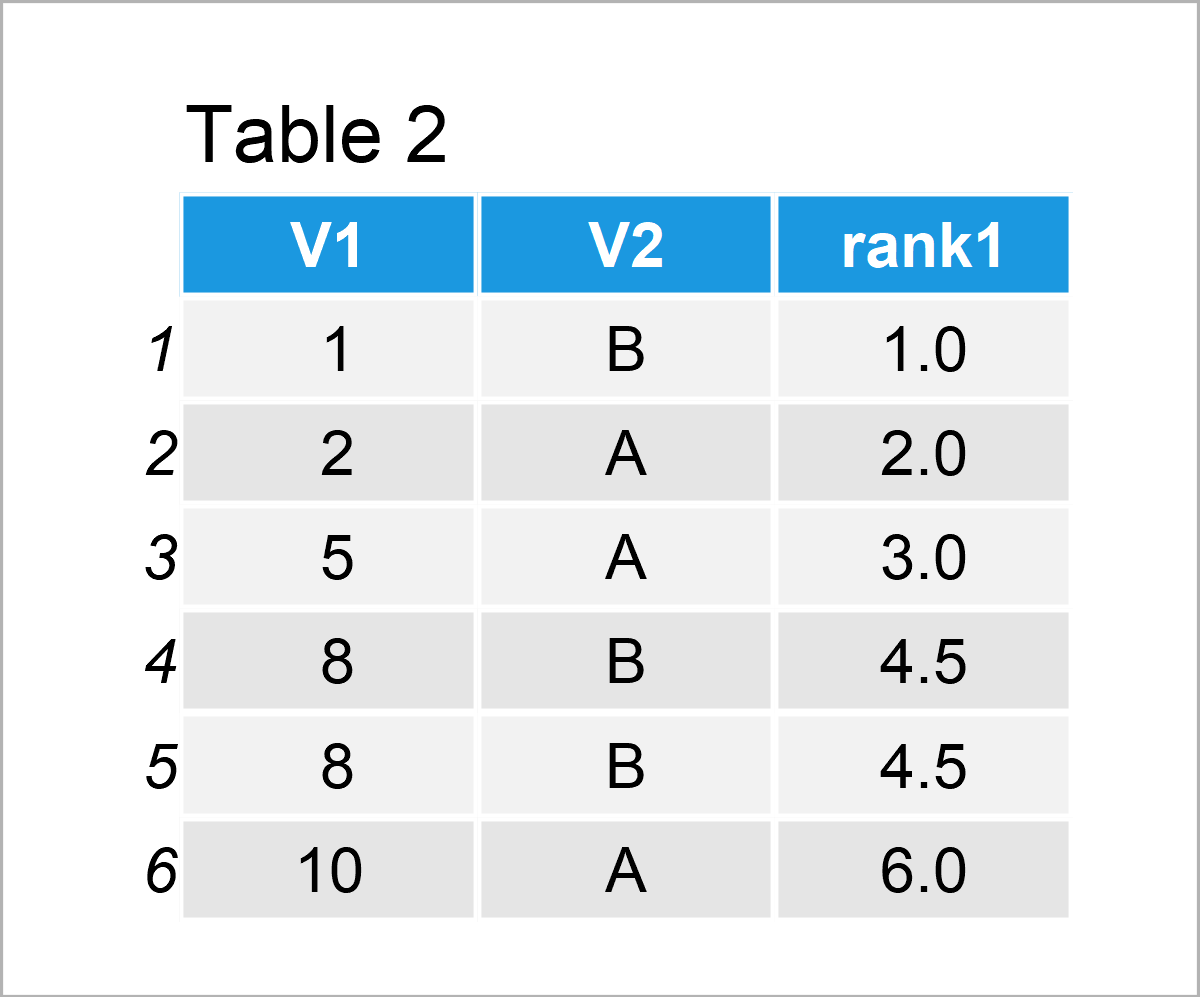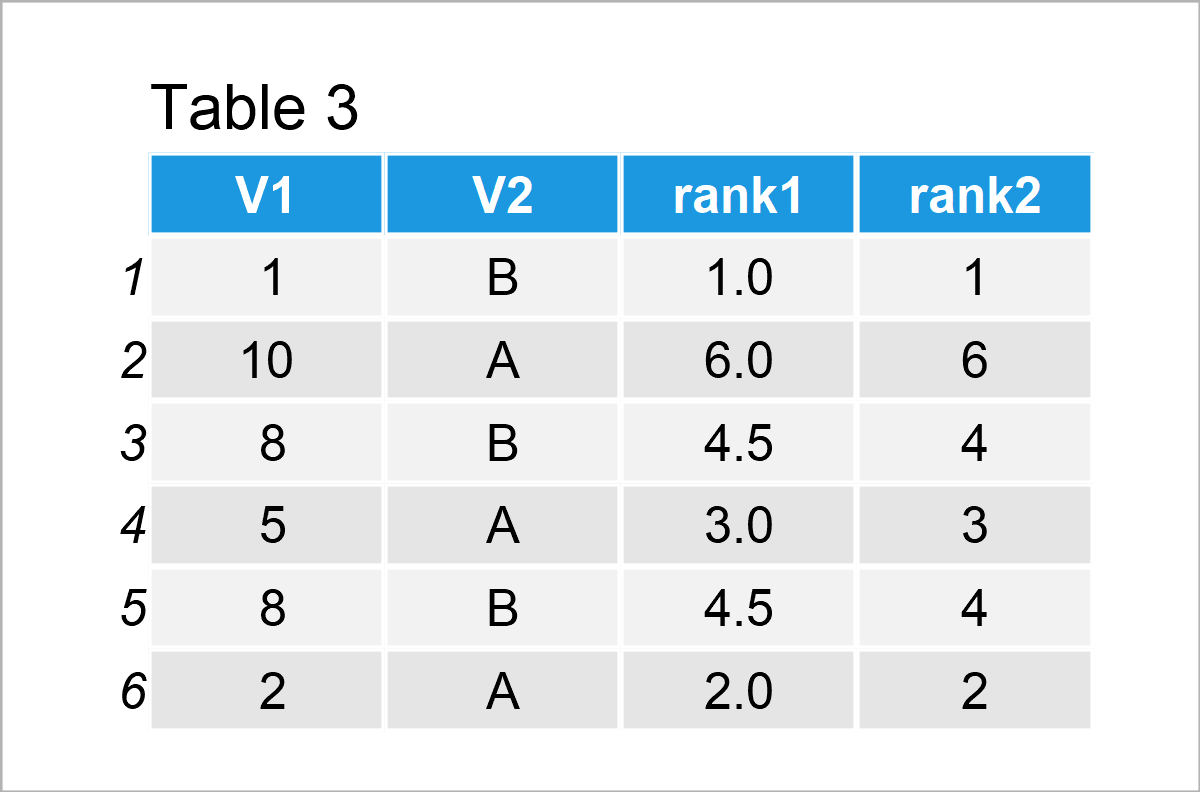# Rank within Groups in R (Example)

This page shows how to get the ranks per group in the R programming language.

The tutorial will contain these content blocks:

So without further ado, let’s dive right in.

## Example Data & Packages

We first have to install and load the data.table package. For more information on the data.table package, see our extra blog post here.

```install.packages("data.table")                                 # Install & load data.table package
library("data.table")```

We also have to create some data that we can use in the example syntax later on:

```D1 <- data.table(V1 = c(1, 10, 8, 5, 8, 2),                    # Generate data
V2 = c("B", "A", "B", "A", "B", "A"))
D1                                                             # Print data```Have a look at the previous table. It shows that our example data contains six rows and two columns. The column V1 has the numeric class and the variable V2 is a character.

## Example: Ranking Options

The following R syntax demonstrates different options of to getting the ranks of the data rows by different columns.

For the ranking, we use function frank from the data.table package.

`?frank`

We first create a variable rank1 containing the ranks of variable V1.

```D1[, rank1 := frank(V1)]                                       # Create ranking variable for V1
D1[order(V1), ]                                                # Reorder data```To make the values of the ranks easier to understand, we have sorted the data according to V1. There are two observations with V1 taking value 8. Therefore – by default in function frank – both observations get assigned the same value, which is the average value of their positions 4 and 5.

Another option is to assign the minimum rank value to those observations with the same rank. That is for our previous example, for ranks 4 and 5 both observations are assigned rank 4.

```D1[, rank2 := frank(V1, ties.method = "min")]                  # Option: What to do about ties in ranking?
D1```Next, we want to see the ranks by group V2. There are different options. In the following, we create rank3 for the rank of all observations according to variables V2 and V1. Furthermore, we create ranking variable rank4 which contains the ranks for each single group member of V2.

```D1[, rank3 := frank(D1, V2, V1, ties.method = "min")]     # Ranking considering V2
D1[, rank4 := frank(V1, ties.method = "min"), by = V2]    # Another ranking option considering V2
D1[order(V2, V1), ]```As shown in Table 4, rank3 contains the ranks according to V2 and V1, rank4 on the other hand counts the ranks for each value of V2.

## Video, Further Resources & Summary

Do you need further details on the R codes of this tutorial? Then you might want to have a look at the following video on my YouTube channel. I’m explaining the R programming syntax of this tutorial in the video.

Furthermore, you may want to read the other articles on this website.

This page was created in collaboration with Anna-Lena Wölwer. Have a look at Anna-Lena’s author page to get further information about her academic background and the other articles she has written for Statistics Globe.

Subscribe to the Statistics Globe Newsletter